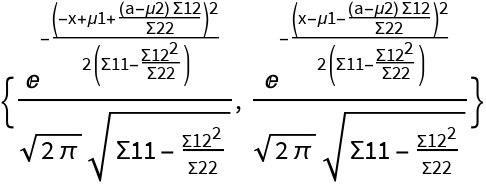#Function Repository Resource:

# ConditionedMultinormalDistribution

Calculate conditional and marginal distributions of the multivariate normal distribution

Contributed by: Sjoerd Smit
 ResourceFunction["ConditionedMultinormalDistribution"][MultinormalDistribution[μ,Σ],i→xi] returns the MultinormalDistribution obtained by conditioning coordinate i to value xi. ResourceFunction["ConditionedMultinormalDistribution"][…,{i1→xi1,i2→xi2,…}] conditions on multiple coordinates. ResourceFunction["ConditionedMultinormalDistribution"][…,{i1,i2,…}→{xi1,xi2,…}] can also be used. ResourceFunction["ConditionedMultinormalDistribution"][…,…,{n1,n2,…}] returns the marginal distributions of coordinates ni after conditioning. ResourceFunction["ConditionedMultinormalDistribution"][Inactive[MultinormalDistribution][…],…] works with SparseArray and SymmetrizedArray objects.

## Details and Options

ResourceFunction["ConditionedMultinormalDistribution"] attempts to fill a gap in the functionality of Conditioned, which currently only works with functions like Probability and Expectation but cannot be used to transform distributions. In particular, Conditioned does not work with TransformedDistribution. Conditioning of distributions is generally non-trivial, but for the MultinormalDistribution, there exist exact analytical formulas.
ResourceFunction["ConditionedMultinormalDistribution"] works with TransformedDistribution. Conditioning of distributions is generally non-trivial, but for the MultinormalDistribution, there exist exact analytical formulas.
As an example, if we have a triple of numbers with joint distribution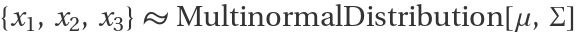, then ResourceFunction["ConditionedMultinormalDistribution"][MultinormalDistribution[μ,Σ],30] is the distribution of the pair {x1,x2} given the knowledge that x3 is equal to 0 (i.e. the distribution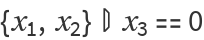).
Calculating the conditioned distribution involves matrix inversion, so for distributions with many coordinates, it is recommended to only request the marginals of interest since computing the full distribution can be expensive.
Like MarginalDistribution, ResourceFunction["ConditionedMultinormalDistribution"][,,{n1,n2,}] will permute the indices of the resulting distribution according the specification {n1,n2,}. For example, the specification {2,1} will swap dimensions 1 and 2.
The specification All can also be used for the marginals.
ResourceFunction["ConditionedMultinormalDistribution"][,,n] returns a NormalDistribution while ResourceFunction["ConditionedMultinormalDistribution"][,,{n}] returns a one-dimensional MultinormalDistribution.
When using , the covariance matrix will not be tested for being positive definite.

## Examples

### Basic Examples

Condition a two-dimensional symbolic multivariate normal distribution on the value of the second coordinate:

 In:=Out=A NormalDistribution is returned if you specify that the first marginal should be computed:

 In:=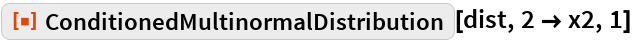Out=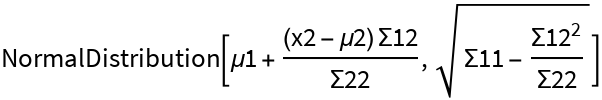Generate a random MultinormalDistribution:

 In:=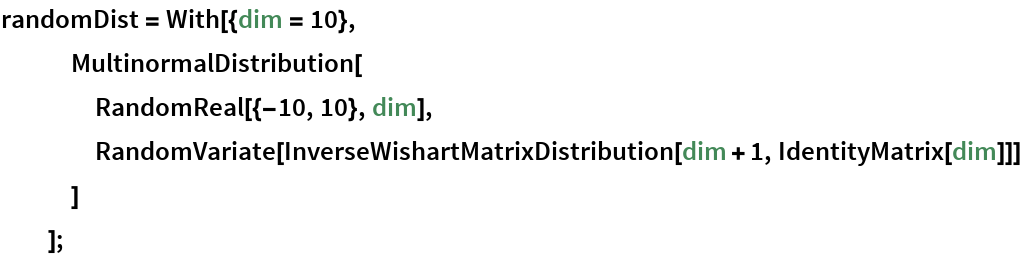Calculate how the expected value of the second coordinate depends on the value of the first:

 In:=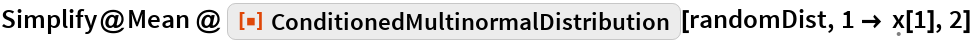Out=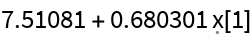Calculate the derivative:

 In:=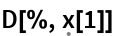Out=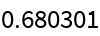Condition on multiple indices and obtain the means of multiple marginals:

 In:=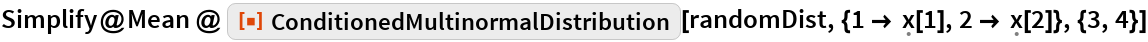Out=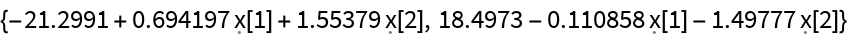### Applications

Generate some x, y data:

 In:=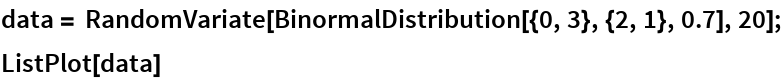Out=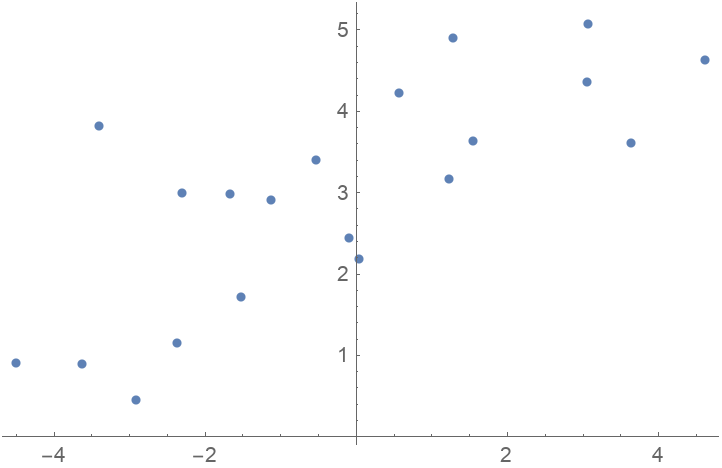Perform a simple linear fit by estimating the joint distribution of the data and then condition it on x:

 In:=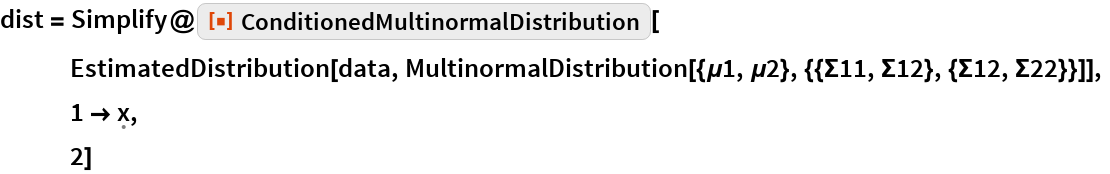Out=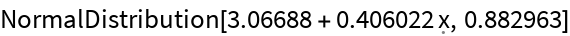Plot the result:

 In:=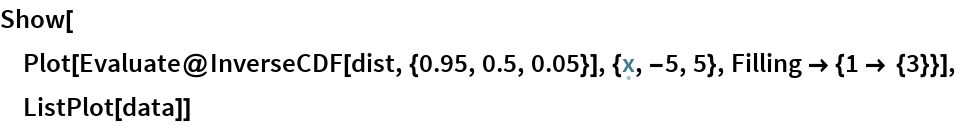Out=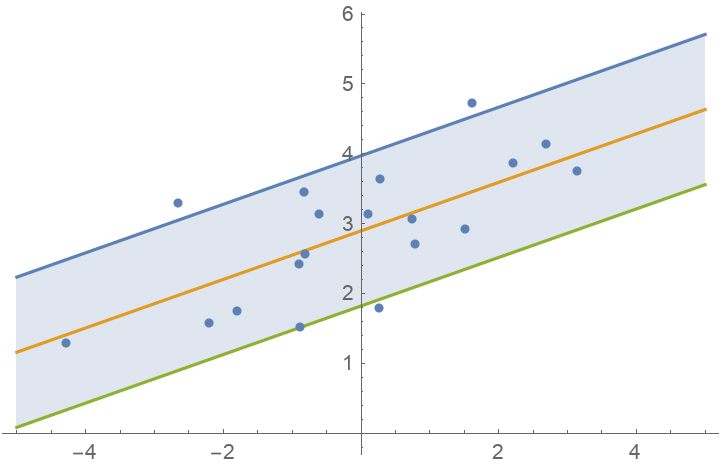### Properties and Relations

MultinormalDistribution normally converts SparseArray and StructuredArray objects to regular lists:

 In:=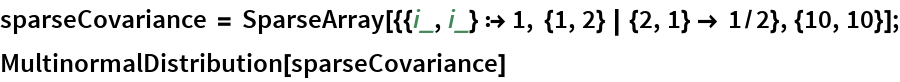Out=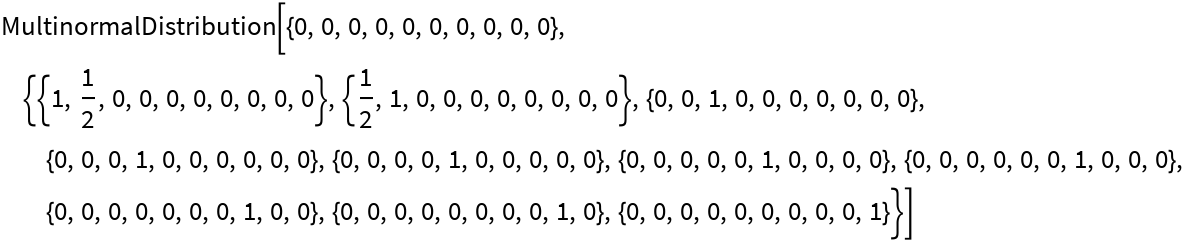Use Inactive to keep the covariance matrix packed as a sparse array:

 In:=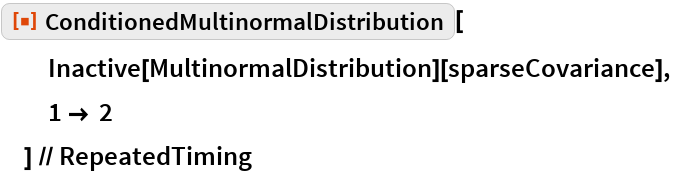Out=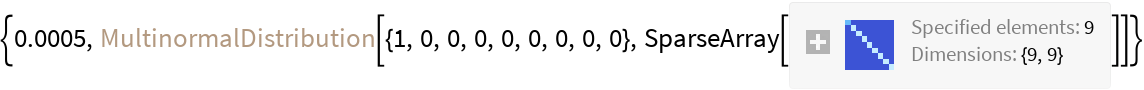This is significantly more computationally efficient than having the covariance unpack into a regular list:

 In:=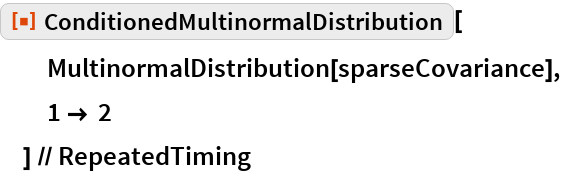Out=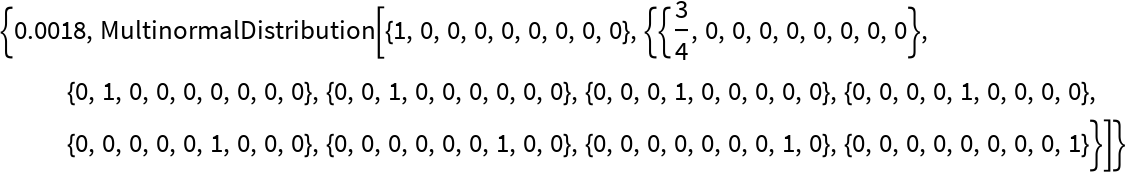Conditioning on variables can be done with Conditioned, but it is less convenient and results in a general ProbabilityDistribution:

 In:=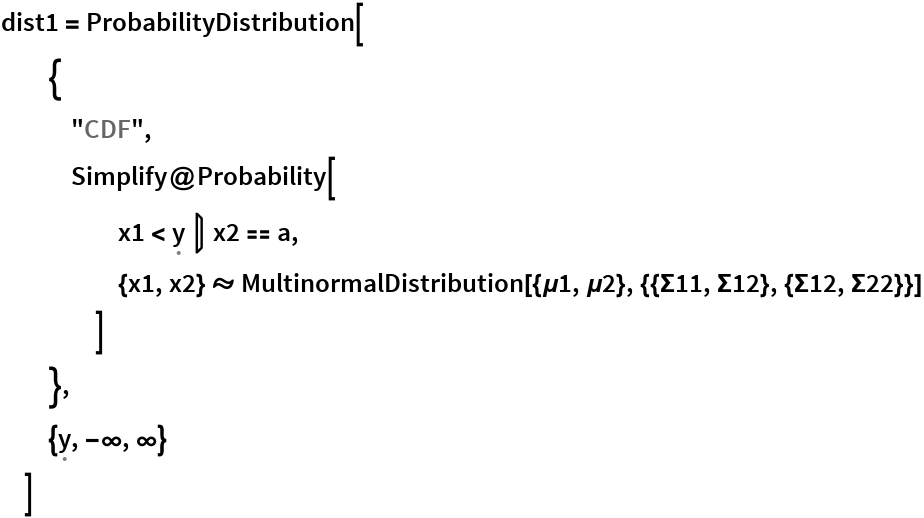Out=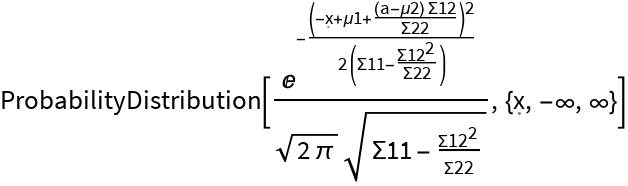The same distribution with ConditionedMultinormalDistribution:

 In:=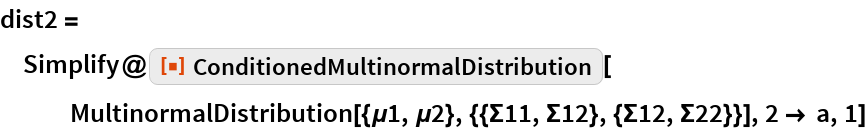Out=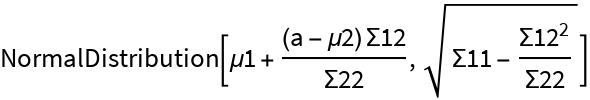The PDFs are the same up to a minus sign under the square:

 In:=Out=